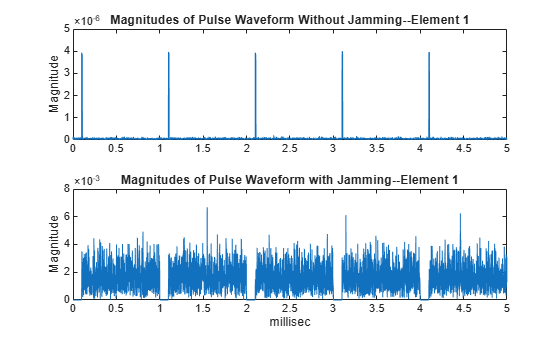## Barrage Jammer

### Support for Modeling Barrage Jammer

The `barrageJammer` object models a broadband jammer. The output of `barrageJammer` is a complex white Gaussian noise sequence. The modifiable properties of the barrage jammer are:

• `ERP` — Effective radiated power in watts

• `SamplesPerFrameSource` — Source of number of samples per frame

• `SamplesPerFrame` — Number of samples per frame

• `SeedSource` — Source of seed for random number generator

• `Seed` — Seed for random number generator

The real and imaginary parts of the complex white Gaussian noise sequence each have variance equal to 1/2 the effective radiated power in watts. Denote the effective radiated power in watts by P. The barrage jammer output is:

`$w\left[n\right]=\sqrt{\frac{P}{2}}x\left[n\right]+j\sqrt{\frac{P}{2}}y\left[n\right]$`

In this equation, `x[n]` and `y[n]` are uncorrelated sequences of zero-mean Gaussian random variables with unit variance.

### Model Barrage Jammer Output

This example examines the statistical properties of the barrage jammer output and how they relate to the effective radiated power (ERP). Create a barrage jammer using an effective radiated power of 5000 watts. Generate output at 500 samples per frame. Then call the `step` function once to generate a single frame of complex data. Using the `histogram` function, show the distribution of barrage jammer output values. The `BarrageJammer` System object uses a random number generator. In this example, the random number generator seed is fixed for illustrative purposes and can be removed.

```rng default jammer = barrageJammer('ERP',5000,... 'SamplesPerFrame',500); y = jammer(); subplot(2,1,1) histogram(real(y)) title('Histogram of Real Part') subplot(2,1,2) histogram(imag(y)) title('Histogram of Imaginary Part') xlabel('Watts')```The mean values of the real and imaginary parts are

`mean(real(y))`
```ans = -1.0961 ```
`mean(imag(y))`
```ans = -2.1671 ```

which are effectively zero. The standard deviations of the real and imaginary parts are

`std(real(y))`
```ans = 50.1950 ```
`std(imag(y))`
```ans = 49.7448 ```

which agree with the predicted value of $\sqrt{ERP/2}$.

### Model Effect of Barrage Jammer on Target Echo

This example demonstrates how to simulate the effect of a barrage jammer on a target echo. First, create the required objects. You need an array, a transmitter, a radiator, a target, a jammer, a collector, and a receiver. Additionally, you need to define two propagation paths: one from the array to the target and back, and the other path from the jammer to the array.

```antenna = phased.ULA(4); Fs = 1e6; fc = 1e9; rng('default') waveform = phased.RectangularWaveform('PulseWidth',100e-6,... 'PRF',1e3,'NumPulses',5,'SampleRate',Fs); transmitter = phased.Transmitter('PeakPower',1e4,'Gain',20,... 'InUseOutputPort',true); radiator = phased.Radiator('Sensor',antenna,'OperatingFrequency',fc); jammer = barrageJammer('ERP',1000,... 'SamplesPerFrame',waveform.NumPulses*waveform.SampleRate/waveform.PRF); target = phased.RadarTarget('Model','Nonfluctuating',... 'MeanRCS',1,'OperatingFrequency',fc); targetchannel = phased.FreeSpace('TwoWayPropagation',true,... 'SampleRate',Fs,'OperatingFrequency', fc); jammerchannel = phased.FreeSpace('TwoWayPropagation',false,... 'SampleRate',Fs,'OperatingFrequency', fc); collector = phased.Collector('Sensor',antenna,... 'OperatingFrequency',fc); amplifier = phased.ReceiverPreamp('EnableInputPort',true);```

Assume that the array, target, and jammer are stationary. The array is located at the global origin, (0,0,0). The target is located at (1000,500,0), and the jammer is located at (2000,2000,100). Determine the directions from the array to the target and jammer.

```targetloc = [1000 ; 500; 0]; jammerloc = [2000; 2000; 100]; [~,tgtang] = rangeangle(targetloc); [~,jamang] = rangeangle(jammerloc);```

Finally, transmit the rectangular pulse waveform to the target, reflect it off the target, and collect the echo at the array. Simultaneously, the jammer transmits a jamming signal toward the array. The jamming signal and echo are mixed at the receiver. Generate waveform

```wav = waveform(); % Transmit waveform [wav,txstatus] = transmitter(wav); % Radiate pulse toward the target wav = radiator(wav,tgtang); % Propagate pulse toward the target wav = targetchannel(wav,[0;0;0],targetloc,[0;0;0],[0;0;0]); % Reflect it off the target wav = target(wav); % Collect the echo wav = collector(wav,tgtang);```

Generate the jamming signal

```jamsig = jammer(); % Propagate the jamming signal to the array jamsig = jammerchannel(jamsig,jammerloc,[0;0;0],[0;0;0],[0;0;0]); % Collect the jamming signal jamsig = collector(jamsig,jamang); % Receive target echo alone and target echo + jamming signal pulsewave = amplifier(wav,~txstatus); pulsewave_jamsig = amplifier(wav + jamsig,~txstatus);```

Plot the result, and compare it with received waveform with and without jamming.

```subplot(2,1,1) t = unigrid(0,1/Fs,size(pulsewave,1)*1/Fs,'[)'); plot(t*1000,abs(pulsewave(:,1))) title('Magnitudes of Pulse Waveform Without Jamming--Element 1') ylabel('Magnitude') subplot(2,1,2) plot(t*1000,abs(pulsewave_jamsig(:,1))) title('Magnitudes of Pulse Waveform with Jamming--Element 1') xlabel('millisec') ylabel('Magnitude')```